This resource function is obsolete. Use the built-in symbol BlockDiagonalMatrix instead.

Function Repository Resource:

# BlockDiagonalMatrix

Create a block-diagonal matrix from submatrices

Contributed by: Bob Sandheinrich
 ResourceFunction["BlockDiagonalMatrix"][m1,m2,…] constructs a matrix with submatrices mi on the diagonal.

## Details and Options

The submatrices mi are included in the specified order.
The submatrices need not be square.
{} and {{}} are treated as 0×0 submatrices.

## Examples

### Basic Examples (2)

Create a block-diagonal matrix from three matrices:

 In:=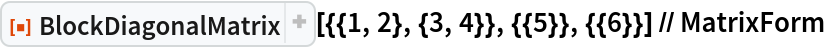Out=Use non-square matrices:

 In:=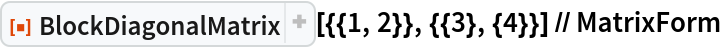Out=### Scope (1)

Empty lists are treated as zero-dimensional matrices:

 In:=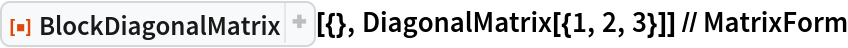Out=### Properties and Relations (1)

The dimensions of the result are the sum of the dimensions of the submatrices:

 In:=Out=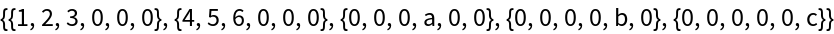In:=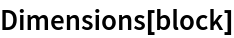Out=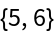In:=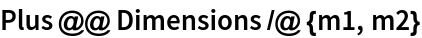Out=## Requirements

Wolfram Language 11.3 (March 2018) or above

## Version History

• 1.0.0 – 13 December 2018